• 数组的定义 数组是相同类型数据的集合 比如全是int 或者全是 string 数组描述是相同类型的数据，他们按照一定的先后次序排序 （先后次序排序，取决于你输入的时候怎么排序） 其中，每一个数据称作一个数组元素...
数组的定义

数组是相同类型数据的集合     比如全是int  或者全是 string

数组描述是相同类型的数据，他们按照一定的先后次序排序
（先后次序排序，取决于你输入的时候怎么排序）

其中，每一个数据称作一个数组元素，每个数据元素可以通过一个下吧来访问。
数组元素是从0开始的，假如数组有10个数字，他最高的下标是9
俗称    从零开始的数组访问

数组声明和 创建
首先必须声明数组变量，才能在程序中使用数组。下面是声明数组变量的语法：
dataType[] arrayRefVar;   //首选的方法
或
dataType[] arrayRefVar[];//效果相同，但不是首选语法

一个是在类型后面加中括号一个是在变量名后面加中括号
package array;

public class ArrayDay01 {
//变量的类型 变量的名字 =  变量的值
//数组类型

public static void main(String[] args) {
int[] nums; //定义    //首选的方法
int nums2 [];  //这2种都是可以的   //效果相同，但不是首选方法
//加一个中括号，就可以代表这是int类型的数组了
//为什么会有2种，是因为int nums2[]是c++类型的，早期是为了让c++的人快速掌握Java。   所以平时就用int[] nums;类型的就可以了

nums = new int;//这里面可以存放10个int类型的数字
}

看一下上面的代码，可以知道int[] nums   和int nums[] 的区别在哪里如果没有数组的话，我们要定义一个数值，就要int x
int x1      int   x2  这样，很麻烦，要写很多代码
比如图中的框框是一个内存，我们定义了一个int x=1  ，那么这个空间就是等于1了， 而那个空间的名称就叫做x
这个空间就是int类型，里面就是数字1 ，但是只能放一个。
要是想要定义10个，就要复制10个，非常麻烦
数组的意思
int[]  nums =  new  int
//int[] nums   数组的意思
//new int   **是新建一个int类型的组，新建了一个空间，空间里面开了10个数值 **    new  是   新   的意思
然后这个空间的名称叫做nums
这个空间里面被开辟了10个空间  ，空间数量多少 取决于new  int 的中括号里面的数值为多少，则空间数量为多少
里面所有的类型都是int类型的
里面的空间，分别对应1-10
然后每个空间都有一个编号也就是所谓的下标
下标是从0开始的
0-9是位置编号，1-10是里面的值
我们要取值的话，就是   nums 下的第0号元素。
相当于原来是一个数，现在是一组数，
但是这一组的同一个名字，所以他里面的类型必须都相同
Java语言使用new操作符来创建组，语法如下
dataType[] arrayRefVar = new dataType[]
//dataType[] arrayRefVar 我们的数组
//dataType[]  为类型
//arrayRefVar  为名称
//new dataType[]   就是新建一个类型为dataType的组

就跟int[] nums = new int[];
这样   int[]  nums  是变量 和 名称
new  int[]  是创建一个类型为int 类型的组
示列
package array;

public class ArrayDay01 {
//变量的类型 变量的名字 =  变量的值
//数组类型
public static void main(String[] args) {
int[] nums; //声明一个数组
nums = new int;//创建一个数组，里面可以存放10个int类型的数字
//给数组元素中赋值
nums = 1;
nums = 2;
nums = 3;
nums = 4;
nums = 5;
nums = 6;
nums = 7;
nums = 8;
nums = 9;
nums = 10;
System.out.println(nums);
}
}

运行一下， 得出1
我们吧0改成9 运行一下，得出10
如果我们吧
nums = 10;
给删掉话，再次运行就会得出一个默认值0
当然也可以
int[] nums = new int[]
//数据类型+上一个中括号代表他是数组
//名称
//在new一个int类型的数组，（中括号里面）再给他分配一个初始的大小
//数组必须给他一个初始值，不然特没有特定的值，万一无线创下去就乱套了
//数组的长度是确定的，一旦创建，它的大小就是不可以更改的

这样，我们吧声明和长度写在一起了
获取数组的长度 语法
arrays.length
​	arrays为数组的名称
length  是获取长度
求数组的全部值的和
package array;
public class ArrayDay01 {
//变量的类型 变量的名字 =  变量的值
//数组类型
public static void main(String[] args) {
int[] nums; //声明一个数组
nums = new int;//创建一个数组，里面可以存放10个int类型的数字
//给数组元素中赋值
nums = 1;
nums = 2;
nums = 3;
nums = 4;
nums = 5;
nums = 6;
nums = 7;
nums = 8;
nums = 9;
nums = 10;
//计算所有元素的和
int sum = 0;
//获取数组长度： arrays.length
for (int i = 0;i<nums.length ; i++){
sum = sum +nums[i];
}
System.out.println("总和为："+sum);
}
}

如图
内存分析

Java内存分析理解

声明一个nums，这个时候在堆里面还是没有东西的。  但是在栈（zhan）里面会压过一个东西，叫做nums.
我们现在只是声明了一个数组，这个数组是空的，他只有一个名字，这个名字并没有什么实际作用
2.第二部创建数组
nums = new int 
new一个int    ，产生了 new这个事件
看上面的图片，new关键词都会放在堆里面
于是乎，在做完这个第二部的时候栈里面的nums
在堆里面做了一件事情
他开辟了一个空间，这个空间就是int类型
里面被分成了10个小块，因为初始的大小为10

声明的时候，这个数组并不存在，只有我们通过new关键词去创建的时候，这个数组才存在，当我们给里面赋值的时候，这里面才有值，没有赋值的话，里面就是默认值

加入我们只有10个元素，那我要是获取第11个元素会怎么样呢？
  //给数组元素中赋值
nums = 1;
nums = 2;
nums = 3;
nums = 4;
nums = 5;
nums = 6;
nums = 7;
nums = 8;
nums = 9;
nums = 10;

System.out.println(nums);

就会看到一个错误java.lang.ArrayIndexOutOfBoundsException
array  数组的意思
IndexOutOfBounds 数组下标越界了
就是说，我们数组只有10个，你却取第11个，
第11个，可能在外面，也有可能不存在，所以是拿不到的
所以就会异常
不过一般来说，我们都是把声明和创建写在一块
int[] nums =new int;

数组的三种初始化

静态初始化

int[] a ={1,2,3};
Man[] mans = {new Man(1,1),new Man(2,2)}


动态初始化

int[] a = new int;
a = 1;
a = 2;


数组的默认初始化

数组是引用类型，它的元素相当于类的实例变量，因此数组一经分配空间，其中的每个元素也被按照实例变量同样的方式被隐式初始化。

静态初始化
 int[] a = {1,2,3,4,5,6,7,8};
System.out.println(a);

我个人理解是
静态初始化
int[]   这是数组的一个类型
a  = 名称
我们之前呢？  是new  int[]  new了一个int让它初始化
我们也可以直接给他初始化，一个{}括号就代表这是一个数组
所有花括号括起来的都是一个数组
然后我们在往数组里面放元素了。放多少个，它的空间就是多大 ，一旦定义之后就不可以改变了
a就是固定那么多
创建的时候顺便赋值
我们输出一下 a   发现等于1
动态初始化
int[] b = new int;
b = 10;
b = 10;
b = 10;
b = 10;
b = 10;
System.out.println(b);
System.out.println(b);
System.out.println(b);
System.out.println(b);
System.out.println(b);

int[] b = new int;

int[]     b         =         new int；
前面的叫声明，后面的叫初始化，给他创建空间
但是空间创建完之后，里面现在的所有值都是零
我们要给他去手动赋值
动态初始化：默认初始化
数组在没有被赋值的时候默认是0，String类型是null     空的意思
 int[] b = new int;
b = 10;
System.out.println(b);
System.out.println(b);

我们运行b和b
发现一个为10   一个为0
没有被初始化的空间，默认值是0
数组的四个基本特点

长度是确定的。数组一旦被创建，它的大小计算不可以改变的。如果说需要改变大小，那么只能重新创建

其中元素的类型必须是相同的类型，不允许出现混合类型
比如里面定义的是int类型，那么就不能出现String类型

数组中的元素可以是任何数据类型，包括基本类型和引用类型。

数组变量属于引用类型，数组也可以看成是对象，数组中的每个元素相当于该对象的成员变量。
数组本身就是对象，Java中对象是在堆中的，因此数组无论保持原始类型还是其他对象类型，数组对象本身是在堆中的
只要使用new关键词，new出来的，以及数组，都是在堆中的

不管是原始类型还是其他对象类型的，所有东西都是保存在堆中的
数组边界

下标的合法区间：[0，lenght-1]，如果越界就会报错;
就比如说，我们的数组长度为10，
但是真正的下标数值为[0,10-1]
就是[0，9]
public stativ void main(String[] args){
int[] a = new int ;
System.out.println(a);
}


上图中的代码，一旦运行就会出现
ArrayIndexOutOfBoundsException:数组下标越界异常！
出现这个错误的时候，就要回去看看，什么地方越界了
示范
package array;

public class ArrayDay02 {
public static void main(String[] args) {
//静态初始化   创建+赋值
int[] a = {1,2,3,4,5,6,7,8};

for(int i = 0; i<a.length; i++){    //a.length 的意思是，可以获取a数组的长度
System.out.println(a[i]);

}

a.length 的意思是
a为我们数组的名称
length为 数组的长度
System.our.println(a[i])；
我们输出一下就会发现全部都运行出来了
我们在i<a.length 的那个地方加个=
for(int i = 0; i<=a.length; i++){
System.out.println(a[i]);

}

再次运行就会发现越界了
i<=8 的时候   i=8   ，但是数组里面没有int 的数组
所以显示越界了
数组边界小结
数组是相同数据类型的有序集合
数组也是对象。因为他是new出来的，数组元素相当于对象的成员变量
数组的长度是确定的，不可以变的，想改变的话，必须重新创建一个
如果越界就会报出   ArrayIndexOutOfBounds
数组使用

For-Each 循环

数组作方法入参

数组作返回值
package array;

public class ArrasyDay03 {
public static void main(String[] args) {
int[] arrays = {1,2,3,4,5};
//打印全部的数组元素
for(int i = 0 ; i< arrays.length ;i++) {
System.out.println(arrays[i]);
}
System.out.println("=========================");

//计算所有元素的和
int sum = 0;
for (int k = 0 ; k<arrays.length;k++){
sum = sum + arrays [k] ;
}
System.out.println("sum :" +sum);        System.out.println("=====================");
//查找最大元素
int max = arrays;
for (int i = 1; i < arrays.length; i++) {
if (arrays[i]>max){
max = arrays[i];
}
}
System.out.println("max ="+max);
}
}


分别是打印数组、求和还有最大元素的写法
For-Each 循环   刚刚我们用的都是普通的for循环
​
public static void main(String[] args) {
int[] arrays = {1,2,3,4,5};

//JDK1.5以上版本    省略了下标   // 用于打印输出
for (int array : arrays) {
//               arrays 代表一个数组
System.out.println(array);
}

我们输入arrays.for 就是自动变成    上面的方法理解就好
for(int array ： arrays)
// int array 是类型+名称
//arrays  是数组的名称
//： 是类似于==的意思

然后我们在输出一下，这样子的for循环是直接吧arrays里面的数值
一个一个打印出来，是比较简单的一种操作
但是还是建议使用这种类型的
for(int i = 0 ; i<arrays.length;i++){
System.out.println(arrays[i]+" ")
}

数组作方法入参  数组作返回值
打印数组元素
 .public static void main(String[] args){
printArray(reverse);
}

public static void printArray(int[] arrays) {
//static  是为了后面方便调用，  就是类变量
//printArray  是方法名称
//int[] arrays   是int类型  arrays名称的数组

for(int i = 0 ;i < arrays.length;i++){
//  arrays.langth    arrays是数组名称
//langth 是长度的意思，  合在一起就是arrays这个数组的长度有多长

System.out.print(arrays[i]+" ");
//print 是运行完之后不换行
}

输出一下，就是反转数组
package array;

public class ArrasyDay04 {
public static void main(String[] args) {
int[] arrays = {1,2,3,4,5};
int[] reverse = reverse(arrays);
printArray(reverse);
}
//反转数组
public static int[] reverse(int[] arrays){
int[] result = new int[arrays.length];
//反转的操作
for (int i = 0, j=result.length-1; i < arrays.length ; i++,j--) {

result[j] = arrays[i];
}
return result;
}
}

第一步
public static int[] reverse(int[] arrays){
//public static  是修饰符
//int[]  为返回的数据类型	  返回一个数组
//reverse  是方法名称   reverse ： 反转
//传递的是数组参数   int[]  arrays    arrays名称的数组类型
//
}

第二部
 public static int[] reverse(int[] arrays){
int[] result = new int[arrays.length];

}

声明并创建一个数组，数值为arrays.length  意思是 arrays数组的长度
这样可以保证，是同一个结果
第三部  反转操作
public static int[] reverse(int[] arrays){
int[] result = new int[arrays.length];
//反转操作
for(int i = 0,j=result.length-1;i< arrays.length;i++,j--){
//j=result.length-1   是因为有个下标是0
result[j] = arrays[i];
//result[j]  因为上面 int[arrays.length];  也就是说他是5
//所以j=result.length-1  =  4  (这是下标)
//而arrats[i]   是从1开始 ，然后递增  到4
//所以j=4  	i=1
//j 是下标符   i是数值
//所以4=1  3=2 2=3 1=4 0=5
//然后排序就是5,4,3,2,1

}
return result;
}

result[j]  因为上面 int[arrays.length];  也就是说他是5
所以j=result.length-1  =  4  (这是下标)
而arrats[i]   是从1开始 ，然后递增  到4
所以j=4  	i=1
j 是下标符   i是数值
所以4=1  3=2 2=3 1=4 0=5
然后排序就是5,4,3,2,1
然后再return返回result的值
然后我们在输出一下
public class ArrasyDay04 {
public static void main(String[] args) {
int[] arrays = {1,2,3,4,5};
int[] reverse = reverse(arrays);
printArray(reverse);


输入reverse(arrays);
就会返回一个结果，这个结果就是已经被反转过的数组
int[] reverse = reverse(arrays);
然后再输出printArray(revers);
运行一下展开全文java
• 然后，我声明了一个属性，类型int数组，通过get set来获取设置前面内部byte数组(set执行函数是将一个int值拆成2个byte值转换后赋值给内部byte数组长度翻一倍) ![set执行函数是将一个int值拆成2个byte值...set 属性 索引 转换
• 2、我怎么知道数组的长度？ 答：'.length'属性获取 3、数组有没有length()这个方法? String有没有length()这个方法 答：数组没有length()这个方法，有length的属性。 String有length()这个方法 4、Java中的任何...
1、java能动态分配数组吗？
答：可以。int i = 12;int[] myInt = new int[i];

2、我怎么知道数组的长度？
答：'.length'属性获取

3、数组有没有length()这个方法? String有没有length()这个方法
答：数组没有length()这个方法，有length的属性。
String有length()这个方法

4、Java中的任何数据类型都可以使用System.out.pritln方法显示
对基本数据类型而言，输出的往往是变量的值；

对于像数组这一类复杂的数据类型，输出的是其堆空间中存储位置的hashCode值

5、操作二维数组的注意点
操作二维数组不应使用常数来控制维数。

具体方法是array.length表示行数，array[row].length来表示row行的列数。

这样当数组行数和列数不相等时，代码可以自动调整为正确的值。

6、显示输出：
String[] stringArray = new String; // 各元素的值默认为null
for (int i = 0; i < stringArray.length; i++) { // 对各元素进行初始化，但没有赋值。
stringArray[i] = new String();
System.out.println(stringArray[i]);
}

答案：空  （有别于null）

7、面试题目：创建一个长度为6的int型数组，要求取值为1-30，同时元素值各不相同
class ArrayExer {
public static void main(String[] args) {
//方式一：
//		int[] arr = new int;
//		for (int i = 0; i < arr.length; i++) {// [0,1) [0,30) [1,31)
//			arr[i] = (int) (Math.random() * 30) + 1;
//
//			boolean flag = false;
//			while (true) {
//				for (int j = 0; j < i; j++) {
//					if (arr[i] == arr[j]) {
//						flag = true;
//						break;
//					}
//				}
//				if (flag) {
//					arr[i] = (int) (Math.random() * 30) + 1;
//					flag = false;
//					continue;
//				}
//				break;
//			}
//		}
//
//		for (int i = 0; i < arr.length; i++) {
//			System.out.println(arr[i]);
//		}
//方式二：
int[] arr = new int;
for (int i = 0; i < arr.length; i++) {// [0,1) [0,30) [1,31)
arr[i] = (int) (Math.random() * 30) + 1;

for (int j = 0; j < i; j++) {
if (arr[i] == arr[j]) {
i--;
break;
}
}
}

for (int i = 0; i < arr.length; i++) {
System.out.println(arr[i]);
}
}
}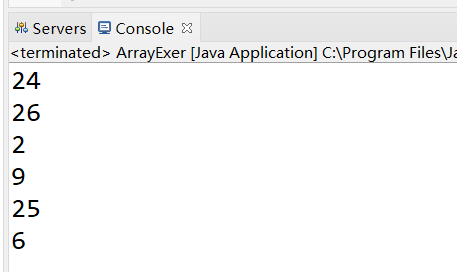8、回形数格式方阵的实现
从键盘输入一个整数（1~20）
则以该数字为矩阵的大小，把1,2,3…n*n 的数字按照顺时针螺旋的形式填入其中。例如： 输入数字2，则程序输出：
	1 2
4 3

输入数字3，则程序输出：
	1 2 3
8 9 4
7 6 5

输入数字4， 则程序输出：
	1   2   3   4
12  13  14  5
11  16  15  6
10   9  8   7

方式一：
class RectangleTest {
public static void main(String[] args) {
Scanner scanner = new Scanner(System.in);
System.out.println("输入一个数字");
int len = scanner.nextInt();
int[][] arr = new int[len][len];

int s = len * len;
/*
* k = 1:向右 k = 2:向下 k = 3:向左 k = 4:向上
*/
int k = 1;
int i = 0, j = 0;
for (int m = 1; m <= s; m++) {
if (k == 1) {
if (j < len && arr[i][j] == 0) {
arr[i][j++] = m;
} else {
k = 2;
i++;
j--;
m--;
}
} else if (k == 2) {
if (i < len && arr[i][j] == 0) {
arr[i++][j] = m;
} else {
k = 3;
i--;
j--;
m--;
}
} else if (k == 3) {
if (j >= 0 && arr[i][j] == 0) {
arr[i][j--] = m;
} else {
k = 4;
i--;
j++;
m--;
}
} else if (k == 4) {
if (i >= 0 && arr[i][j] == 0) {
arr[i--][j] = m;
} else {
k = 1;
i++;
j++;
m--;
}
}
}

// 遍历
for (int m = 0; m < arr.length; m++) {
for (int n = 0; n < arr[m].length; n++) {
System.out.print(arr[m][n] + "\t");
}
System.out.println();
}
}
}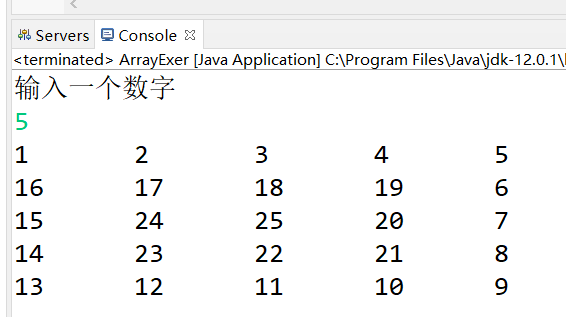方式二：
class RectangleTest1 {

public static void main(String[] args) {
int n = 7;
int[][] arr = new int[n][n];

int count = 0; // 要显示的数据
int maxX = n - 1; // x轴的最大下标
int maxY = n - 1; // Y轴的最大下标
int minX = 0; // x轴的最小下标
int minY = 0; // Y轴的最小下标
while (minX <= maxX) {
for (int x = minX; x <= maxX; x++) {
arr[minY][x] = ++count;
}
minY++;
for (int y = minY; y <= maxY; y++) {
arr[y][maxX] = ++count;
}
maxX--;
for (int x = maxX; x >= minX; x--) {
arr[maxY][x] = ++count;
}
maxY--;
for (int y = maxY; y >= minY; y--) {
arr[y][minX] = ++count;
}
minX++;
}

for (int i = 0; i < arr.length; i++) {
for (int j = 0; j < arr.length; j++) {
String space = (arr[i][j] + "").length() == 1 ? "0" : "";
System.out.print(space + arr[i][j] + " ");
}
System.out.println();
}
}
}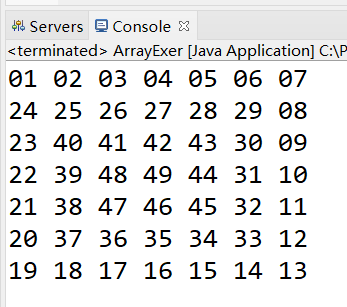9、下面数组定义正确的有：
A.String strs[] = { ‘a’ ‘b’ ‘c’};
B.String[] strs = {“a”, “b”, “c”};
C.String[] strs = new String{“a” ”b” ”c”};
D.String strs[] = new String[]{“a”, “b”, “c”};
E.String[] strs = new String{“a”, “b”, “c”};

答案：
B_D
10、写出结果
class Demo{
public static void main(String[] args){
String foo="blue";
boolean[] bar=new boolean;
if(bar){
foo="green";
}
System.out.println(foo);
}
}

[输出]：blue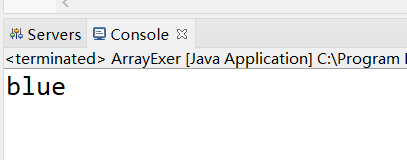11、练习Arrays工具类的使用：
1、从键盘输入本组学员的成绩，放到数组中
2、用for循环显示所有学员的成绩
3、排序：从低到高
4、查找是否有正好60分的，如果有返回位置
5、复制成绩最低三名构成新数组
6、用工具类打印成绩最低三名成绩
/*
练习：
1、从键盘输入本组学员的成绩，放到数组中
2、用foreach显示所有学员的成绩
3、排序：从低到高
4、查找是否有正好60分的，如果有返回位置
5、复制成绩最低三名构成新数组
6、用工具类打印成绩最低三名成绩
*/
import java.util.Scanner;
import java.util.Arrays;

class TestArraysExer{
public static void main(String[] args){
//1、声明一个数组并创建一个数组
int[] scores = new int;

//2、从键盘输入成绩
Scanner input = new Scanner(System.in);
for(int i=0; i<scores.length; i++){
//成绩存在数组的元素中
//为元素赋值
System.out.print("请输入第" + (i+1) + "个学员的成绩:");
scores[i] = input.nextInt();
}

//3、显示成绩
//用foreach显示所有学员的成绩
System.out.println("本组学员的成绩如下：");
for(int s = 0; s < scores.length;s++){
System.out.println(scores[s]);
}
//4、排序：从低到高
Arrays.sort(scores);

System.out.println("排序后的结果：" + Arrays.toString(scores));

//5、查找60分
int index = Arrays.binarySearch(scores, 60);
if(index<0){
System.out.println("没有正好60分的");
}else{
System.out.println("60分的索引位置：" + index);
}

//6、复制成绩最低三名构成新数组
//int[] newArray = Arrays.copyOfRange(scores, 0, 3);
int[] newArray = Arrays.copyOf(scores,  3);

//7、用工具类打印成绩最低三名成绩
System.out.println("成绩最低的三名同学是：" +  Arrays.toString(newArray));
}
}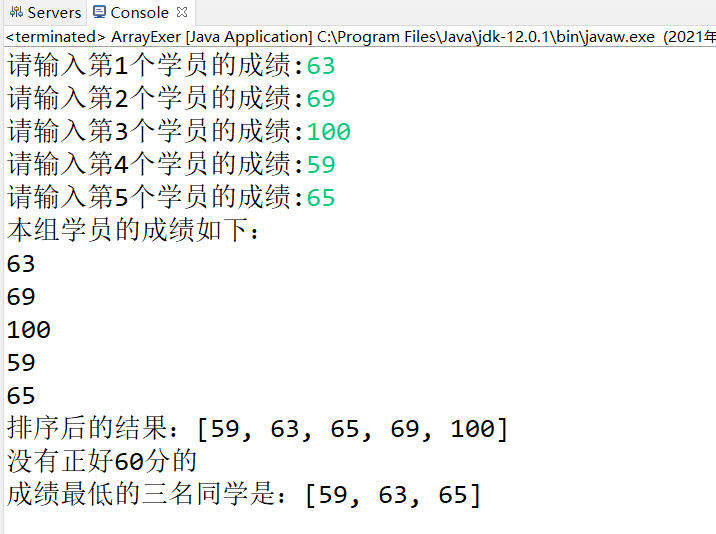12、一维数组a[]，用java代码将数组元素顺序颠倒
public static void main(String[] args) {
int[] a = new int[] { (int) (Math.random() * 1000),
(int) (Math.random() * 1000), (int) (Math.random() * 1000),
(int) (Math.random() * 1000), (int) (Math.random() * 1000) };

System.out.println(a);
System.out.println(Arrays.toString(a));
swap(a);
System.out.println(Arrays.toString(a));
}

public static void swap(int a[]) {
int len = a.length;
for (int i = 0; i < len / 2; i++) {
int tmp = a[i];
a[i] = a[len - 1 - i];
a[len - 1 - i] = tmp;
}
}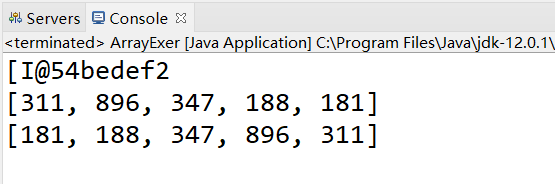展开全文java 数据结构 算法
• //1）创建一个长度为5类型为学生Student一维数组 //2）键盘输入5个学生信息 //3）调用Score类中静态方法GetMax得到成绩最高学生对象 //4）通过for循环遍历出一维数组中成绩为最高成绩所有学 ...c#
• dll中函数描述  function SendRcvB...看到调用需要给 传出参数分配内存空间，java应该怎么写呢定义一个空byte数组吗？ 里边只给了Delphi例子 GetMem(pDataOutput, 4096); 请问这个用java怎么c++ java
• 怎么知道数组的长度？ .length属性获取 数组有没有length()这个方法? String有没有length()这个方法 答：数组没有length()这个方法，有length的属性。String有length()这个方法 Java中的任何数据类型都可...
章节练习题及面试题
简答题：
java能动态分配数组吗？
答：可以。int i = 12;int[] myInt = new int[i];
我怎么知道数组的长度？
.length属性获取
数组有没有length()这个方法? String有没有length()这个方法
答：数组没有length()这个方法，有length的属性。String有length()这个方法
Java中的任何数据类型都可以使用System.out.pritln方法显示
对基本数据类型而言，输出的往往是变量的值；
对于像数组这一类复杂的数据类型，输出的是其堆空间中存储位置的hashCode值
操作二维数组的注意点
操作二维数组不应使用常数来控制维数。具体方法是array.length表示行数，
array[row].length来表示row行的列数。这样当数组行数和列数不相等时，
代码可以自动调整为正确的值。
编程题：
显示输出
String[] stringArray = new String; // 各元素的值默认为null
for (int i = 0; i < stringArray.length; i++) { // 对各元素进行初始化，但没有赋值。
stringArray[i] = new String();
System.out.println(stringArray[i]);
}
答案：空  （有别于null）
面试题目：创建一个长度为6的int型数组，要求取值为1-30，同时元素值各不相同
class ArrayExer {
public static void main(String[] args) {
//方式一：
//		int[] arr = new int;
//		for (int i = 0; i < arr.length; i++) {// [0,1) [0,30) [1,31)
//			arr[i] = (int) (Math.random() * 30) + 1;
//
//			boolean flag = false;
//			while (true) {
//				for (int j = 0; j < i; j++) {
//					if (arr[i] == arr[j]) {
//						flag = true;
//						break;
//					}
//				}
//				if (flag) {
//					arr[i] = (int) (Math.random() * 30) + 1;
//					flag = false;
//					continue;
//				}
//				break;
//			}
//		}
//
//		for (int i = 0; i < arr.length; i++) {
//			System.out.println(arr[i]);
//		}
//方式二：
int[] arr = new int;
for (int i = 0; i < arr.length; i++) {// [0,1) [0,30) [1,31)
arr[i] = (int) (Math.random() * 30) + 1;
			for (int j = 0; j < i; j++) {
if (arr[i] == arr[j]) {
i--;
break;
}
}
}

for (int i = 0; i < arr.length; i++) {
System.out.println(arr[i]);
}
}

}
回形数格式方阵的实现
从键盘输入一个整数（1~20）
则以该数字为矩阵的大小，把1,2,3…n*n 的数字按照顺时针螺旋的形式填入其中。例如： 输入数字2，则程序输出： 1 2
4 3
输入数字3，则程序输出： 1 2 3
8 9 4
7 6 5
输入数字4， 则程序输出：
1   2   3   4
12  13  14  5
11  16  15  6
10   9  8    7
方式一：
class RectangleTest {
public static void main(String[] args) {
Scanner scanner = new Scanner(System.in);
System.out.println(“输入一个数字”);
int len = scanner.nextInt();
int[][] arr = new int[len][len];
	int s = len * len;
/*
* k = 1:向右 k = 2:向下 k = 3:向左 k = 4:向上
*/
int k = 1;
int i = 0, j = 0;
for (int m = 1; m <= s; m++) {
if (k == 1) {
if (j < len && arr[i][j] == 0) {
arr[i][j++] = m;
} else {
k = 2;
i++;
j--;
m--;
}
} else if (k == 2) {
if (i < len && arr[i][j] == 0) {
arr[i++][j] = m;
} else {
k = 3;
i--;
j--;
m--;
}
} else if (k == 3) {
if (j >= 0 && arr[i][j] == 0) {
arr[i][j--] = m;
} else {
k = 4;
i--;
j++;
m--;
}
} else if (k == 4) {
if (i >= 0 && arr[i][j] == 0) {
arr[i--][j] = m;
} else {
k = 1;
i++;
j++;
m--;
}
}
}

// 遍历
for (int m = 0; m < arr.length; m++) {
for (int n = 0; n < arr[m].length; n++) {
System.out.print(arr[m][n] + "\t");
}
System.out.println();
}
}

}
方式二：
class RectangleTest1 {
public static void main(String[] args) {
int n = 7;
int[][] arr = new int[n][n];

int count = 0; // 要显示的数据
int maxX = n - 1; // x轴的最大下标
int maxY = n - 1; // Y轴的最大下标
int minX = 0; // x轴的最小下标
int minY = 0; // Y轴的最小下标
while (minX <= maxX) {
for (int x = minX; x <= maxX; x++) {
arr[minY][x] = ++count;
}
minY++;
for (int y = minY; y <= maxY; y++) {
arr[y][maxX] = ++count;
}
maxX--;
for (int x = maxX; x >= minX; x--) {
arr[maxY][x] = ++count;
}
maxY--;
for (int y = maxY; y >= minY; y--) {
arr[y][minX] = ++count;
}
minX++;
}

for (int i = 0; i < arr.length; i++) {
for (int j = 0; j < arr.length; j++) {
String space = (arr[i][j] + "").length() == 1 ? "0" : "";
System.out.print(space + arr[i][j] + " ");
}
System.out.println();
}
}

}
下面数组定义正确的有：
A.String strs[] = { ‘a’ ‘b’ ‘c’};
B.String[] strs = {“a”, “b”, “c”};
C.String[] strs = new String{“a” ”b” ”c”};
D.String strs[] = new String[]{“a”, “b”, “c”};
E.String[] strs = new String{“a”, “b”, “c”};
答案：
B_D
写出结果
class Demo{
public static void main(String[] args){
String foo=“blue”;
boolean[] bar=new boolean;
if(bar){
foo=“green”;
}
System.out.println(foo);
}
}
[输出]：blue
下面哪个数组定义是错误的
并对错误的答案加上单行注释，写出错误的原因。
A，float[]=new float; //
B, float f2[]=new float[];//
C, float[] f1=new float;//
D, boolean[] b={“true”,“false”,“true”};//
E, double f4[]={1,3,5}; //
F, int f5[]=new int{2,3,4}; //
G, float f4[]={1.2F,3.0,5.4};//
Arrays工具类的使用
练习：
1、从键盘输入本组学员的成绩，放到数组中
2、用for循环显示所有学员的成绩
3、排序：从低到高
4、查找是否有正好60分的，如果有返回位置
5、复制成绩最低三名构成新数组
6、用工具类打印成绩最低三名成绩
/*
练习：
1、从键盘输入本组学员的成绩，放到数组中
2、用foreach显示所有学员的成绩
3、排序：从低到高
4、查找是否有正好60分的，如果有返回位置
5、复制成绩最低三名构成新数组
6、用工具类打印成绩最低三名成绩
*/
import java.util.Scanner;
import java.util.Arrays;
class TestArraysExer{
public static void main(String[] args){
//1、声明一个数组并创建一个数组
int[] scores = new int;
	//2、从键盘输入成绩
Scanner input = new Scanner(System.in);
for(int i=0; i<scores.length; i++){
//成绩存在数组的元素中
//为元素赋值
System.out.print("请输入第" + (i+1) + "个学员的成绩:");
scores[i] = input.nextInt();
}

//3、显示成绩
//用foreach显示所有学员的成绩
System.out.println("本组学员的成绩如下：");
for(int s = 0; s < scores.length;i++){
System.out.println(scores[s]);
}
//4、排序：从低到高
Arrays.sort(scores);

System.out.println("排序后的结果：" + Arrays.toString(scores));

//5、查找60分
int index = Arrays.binarySearch(scores, 60);
if(index<0){
System.out.println("没有正好60分的");
}else{
System.out.println("60分的索引位置：" + index);
}

//6、复制成绩最低三名构成新数组
//int[] newArray = Arrays.copyOfRange(scores, 0, 3);
int[] newArray = Arrays.copyOf(scores,  3);

//7、用工具类打印成绩最低三名成绩
System.out.println("成绩最低的三名同学是：" +  Arrays.toString(newArray));
}

}
数组赋值练习1
创建一个char类型的26个元素的数组，分别 放置’A’-‘Z’。
使用for循环访问所有元素并打印出来。
数组赋值练习2
创建一个char类型的36个元素的数组，前26个元素放置’A’-‘Z’,   后10个元素放置’0’-‘9’。
使用for循环访问所有元素并打印出来。
提示：char类型数据运算 ‘A’+1 -> ‘B’，‘0’+1 -> ‘1’
一维数组a[]，用java代码将数组元素顺序颠倒
public static void main(String[] args) {
int[] a = new int[] { (int) (Math.random() * 1000),
(int) (Math.random() * 1000), (int) (Math.random() * 1000),
(int) (Math.random() * 1000), (int) (Math.random() * 1000) };
	System.out.println(a);
System.out.println(Arrays.toString(a));
swap(a);
System.out.println(Arrays.toString(a));
}

public static void swap(int a[]) {
int len = a.length;
for (int i = 0; i < len / 2; i++) {
int tmp = a[i];
a[i] = a[len - 1 - i];
a[len - 1 - i] = tmp;
}

}
任意一个人输入年龄，就可以判断出他是哪个年龄段的人？
/*
0-9   儿童
10-19 少年
20-29 青少年
30-39 青年
40-49 壮年
50-59 中年
60-69 中老年
70-79 老年
80-89 老老年
90-99 老老老年
*/
//1.switch
int age = 34;
switch(age/10){
case 0:		…0-9…
case 1:   …10-19…
case 2:   …
}
//2.if…else…
if(age>=0 && age<10){
…
}else if(age>=10 && age< 20){
…
}else…
	//3.数据结构的思想
int age = 22;
String[] arr = {"儿童","少年","青少年","青年","壮年","中年","","","",""};
int idx = age/10;

System.out.println(arr[idx]);
用数组求出斐波那契数列的前20项值
对10个整数进行按照从小到大的顺序排序
求一个3*3矩阵对角线元素之和
<提示>
程序分析：利用双重for循环控制输入二维数组，再将a[i][i]累加后输出。
有一个已经排好序的数组。现输入一个数，要求按原来的规律将它插入数组中。
<提示>
程序分析：首先判断此数是否大于最后一个数，然后再考虑插入中间的数的情况，插入后此元素之后的数，依次后移一个位置。
利用随机数生成一个整数数组，数组中有10个元素，每个元素的值都在1-30之间，且要求各个数值不能相同。打印该数组。
将一个数组逆序输出。
<提示>程序分析：用第一个与最后一个交换。
输入数组，最大的与第一个元素交换，最小的与最后一个元素交换，输出数组。
有n个整数，使其前面各数顺序向后移m个位置，最后m个数变成最前面的m个数
定义一个4行4列的二维数组，逐个从键盘输入值，然后将第1行和第4行的数据进行交换，将第2行和第3行的数据进行交换
定义一个3行4列的二维数组，逐个从键盘输入值，编写程序将四周的数据清0
定义一个3行4列的二维数组，逐个从键盘输入值，将左下三角的值清0
定义一个4行4列的二维数组，逐个从键盘输入值，将对角线的值清0
定义一个N*N二维数组，从键盘上输入值，找出每行中最大值组成一个一维数组并输出

`
展开全文java
• //对于结构体中指针数组，一般采用IntPtr数组 [MarshalAs(UnmanagedType.LPStruct)] public S_BR_DATETIME stModifyDateTime; //对于结构体中指针数组，一般采用IntPtr数组 } 使用Marshal.SizeOf...
• 问题出在isize：文档描述：[in] 传入一个无符号双字节长度为22 的数组，且 isize和isize为传入图像宽度，isize和 isize为传入图像高度 这isize怎么提前定义？ 我尝试使用isize = bytearray()处理...ctypes python
• ## 你必须知道的495个C语言问题

千次下载 热门讨论 2015-05-08 11:09:25
4.4　我用指针操作int数组的时候遇到了麻烦。 4.5　我有一个char*型指针碰巧指向一些int型变量，我想跳过它们。为什么((int*)p)++;这样的代码不行？ 4.6　为什么不能对void*指针进行算术操作？ 4.7　我有些解析...C语言
• 4.4　我用指针操作int数组的时候遇到了麻烦。　46 4.5　我有一个char *型指针碰巧指向一些int型变量，我想跳过它们。为什么((int *)p)++; 这样的代码不行？　47 4.6　为什么不能对void *指针进行算术操作？　47 4.7...
• 4.4　我用指针操作int数组的时候遇到了麻烦。　46 4.5　我有一个char *型指针碰巧指向一些int型变量，我想跳过它们。为什么((int *)p)++; 这样的代码不行？　47 4.6　为什么不能对void *指针进行算术操作？　47 4.7...C 进阶
• 很多人初学程序时，总是在想，那么多算法该怎么写呀？那么多数据结构都不熟悉，该怎么实现呀？总是担心英语不好程序学不精通，数学不好写程序无法达到巅峰。学程序越多，不懂知识越多。 这种想法很正常，...
• 在上一步讲解BeginRead函数时候还有一个入口参数state，如果我们传递了buff进去，那么，在这里我们要强制转换成byte[]类型byte[] data= (byte[])result.AsyncState，转换完毕后，我们还要获取缓冲区大小int ...
• 在上一步讲解BeginRead函数时候还有一个入口参数state，如果我们传递了buff进去，那么，在这里我们要强制转换成byte[]类型byte[] data= (byte[])result.AsyncState，转换完毕后，我们还要获取缓冲区大小int ...SOCKET源码
• * 获取当前步数数字高度 * * @param fontSize 字体大小 * @return 字体高度 */ public int getFontHeight(float fontSize) { Paint paint = new Paint(); paint.setTextSize(fontSize); Rect bounds_...
• 4.6.3 引用类型数组的初始化 96 4.6.4 没有多维数组 99 学生提问：我是否可以让图4.13中灰色覆盖的数组元素再次指向另一个数组？这样不可以扩展成三维数组吗？甚至扩展到更多维的数组？ 101 4.6.5 操作数组的工具...
• [MarshalAs(UnmanagedType.ByValTStr, SizeConst = 2)] //这里2就是数组长度 public string resp_code; /*卡号 (20字节，左对齐，不足部分补空格)*/ [MarshalAs(UnmanagedType.ByValTStr, SizeConst = 20)]...c#
• 1.3.5 给定一个整数数组和一个整数，返回两个数组的索引，这两个索引指向的数字的加和等于指定的整数。需要最优的算法，分析算法的空间和时间复杂度 1.3.6 假如给你一个新产品，你将从哪些方面来保障它的质量？ ...
• 163 理解指向数组的指针 164 访问数组元素 165 理解数组运算 166 递增和递减数组变量 167 声明多维数组 第十五章 宏、常量和预处理命令：定义及使用常量 168 理解常量 169 使用预处理命令#define 170 注意#define...
• 163 理解指向数组的指针 164 访问数组元素 165 理解数组运算 166 递增和递减数组变量 167 声明多维数组 第十五章 宏、常量和预处理命令：定义及使用常量 168 理解常量 169 使用预处理命令#define 170 注意#define...
• 163 理解指向数组的指针 164 访问数组元素 165 理解数组运算 166 递增和递减数组变量 167 声明多维数组 第十五章 宏、常量和预处理命令：定义及使用常量 168 理解常量 169 使用预处理命令#define 170 注意#define...
• 163 理解指向数组的指针 164 访问数组元素 165 理解数组运算 166 递增和递减数组变量 167 声明多维数组 第十五章 宏、常量和预处理命令：定义及使用常量 168 理解常量 169 使用预处理命令#define 170 注意#define...
• Int是java原始数据类型，Integer是java为int提供封装类。Java为每个原始类型提供了封装类。 原始类型封装类 booleanBoolean charCharacter byteByte shortShort intInteger longLong floatFloat doubleDouble ...